##### Solid Model Nodes versus Voxels

A solid or block model ("RwMod" file) is a 3-dimensional grid of imaginary lines that overlays your source data points (shown below in red).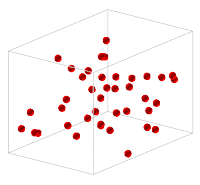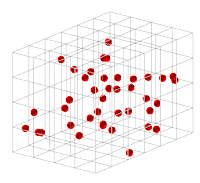In the process of modeling, the program assigns a value to the line intersections, called nodes, shown below as black dots.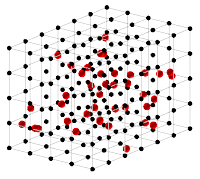Each node has an X,Y, Z location, and a "G" value that the program interpolates.  The G value can represent analytical values, geophysical measurements, lithology materials, etc.  See Solid Modeling Methods for more information about how the interpolation takes place.

The dimensions of your solid models are generally established in your Output Dimensions settings, though they can also be defined manually.  The dimensions of a solid model are illustrated in the diagram shown below.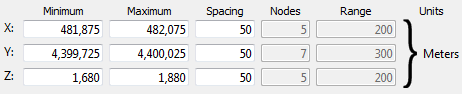These items define the dimensions of a solid model:

• X-Minimum: The westernmost node location.
• X-Maximum: The easternmost node location.
• X-Spacing: The spacing, in your map units, between nodes along the x axis.
• Y-Minimum: The southernmost node location.
• Y-Maximum: The northernmost node location.
• Y-Spacing: The spacing, in your map units, between nodes along the y axis.
• Z-Minimum: The lowest node location.
• Z-Maximum: The highest node location.
• Z-Spacing: the spacing, in your elevation units, between nodes along the z axis.

In this example, there are only 5 nodes west-to-east, 7 nodes south-to-north, and 5 nodes bottom-to-top in the project space (a very coarse model). The volume of this node space is 200 m x 300 m x 200 m = 12,000,000 cubic meters.

However, when RockWorks interpolates a solid model, it creates a voxel or 3D cell around each node, so that the extent of the model actually increases. Here's a view of semi-transparent voxels with the nodes displayed in the center of each voxel.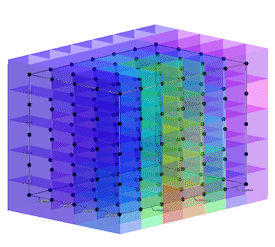The volume of this solid model is larger than the node space by half a voxel along each edge of the block. The model volume is computed by calculating the volume of each voxel (50m * 50m * 50m = 125,000 cubic m) times the number of voxels (5 * 7 * 5 = 175) = 21,875,000 cubic m. In this example, the difference between the node space volume versus model volume is large because the model is coarse (voxels are few and large).

The discrepancy between the node space and the model space will decrease as you increase the density of your model, since the half-a-voxel bulge will be much smaller. Let's say you tightened up the node spacing to 10 m: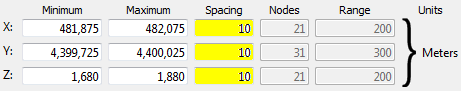Here's a picture of the nodes and voxels with the tighter spacing: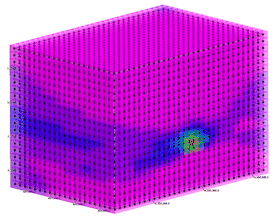In this example, the node space is still 200 m x 300 m x 200 m = 12,000,000 cubic meters. But the model volume is much smaller: the individual voxel volume (10m x 10m x 10m = 1000 cubic m) times the number of voxels (21 x 31 x 21 = 13671) = 13,671,000 cubic m.

So... if it is important that the solid model volume complies with your project boundaries, then you need to be sure that the node extents are set a half a voxel distance inside the project boundaries.

See also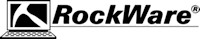RockWare home page